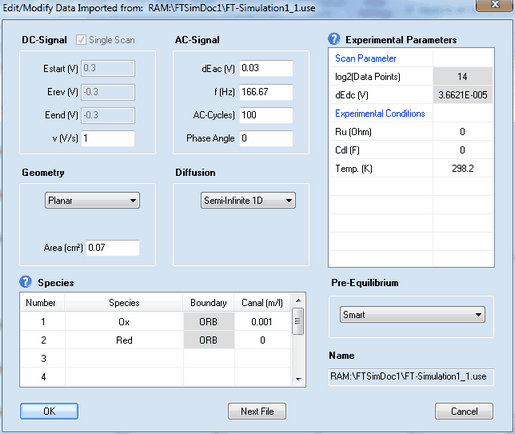﻿ Menu Command: Edit/Modify
DigiElch Help

# Menu Command: Edit/Modify# Menu Command: Edit/ModifyThis command opens a dialog box that enables the user to view (or modify) the parameters imported from an experimental data file.Most of the parameters have exactly the same meaning as already described for cyclic voltammetry. The meaning and functionality of the remaining parameter is as follows:

## 1. DC - Signal

Check Box: Single Scan
This check box is activated if only the forward scan of the DC-signal has been executed. Eend (V) is automatically set equal to Erev(V) and the input field for Eend (V) will be blocked.

Estart (V), Erev, Eend (V), v(s)
The DC-scan can be composed of up to 2 scan segments characterized by starting potential, Estart (V), end-potential, Eend (V), and scan rate, v (V/s).

## 2. Scan Parameters

log2(Data Points)
The number of data points used for generating the signal. It must be an integer power of 2 and is 2^14 = 16384 by default.

dEdc (V)
The potential difference between subsequent data points (fitting the selected number of Data Points) is shown in the (read only) parameter dEdc(V).

## 3. AC - Signal

dEac (V), f(Hz), Phase Angle
The superimposed AC-signal is characterized by Amplitude, dEac (V), frequency, f(Hz), and Phase Angle. The frequency is adjusted such as to ensure an integer value for the number of AC-Cycles.

AC-Cycles
The integer number of AC-Cycles which is superimposed to the DC-signal.